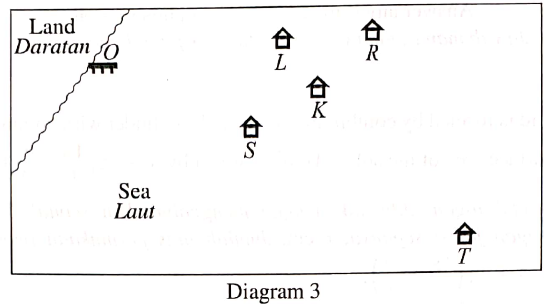# Kelongs (SPM Additional Mathematics 2019)

Solution by scale drawing is not accepted.
Diagram 3 shows the positions of jetty $O$ and kelongs $K$, $L$, $R$, $S$, and $T$ in the sea.Kelong $L$ is situated $400 \mathrm{m}$ from jetty $O$ and kelong $R$ is situated $600 \mathrm{m}$ from jetty $O$ in the direction of $OL$. Kelong $S$ is situated $300 \mathrm{m}$ from jetty $O$ and kelong $T$ is situated $600 \mathrm{m}$ from kelong $S$ in the direction of $OS$. Kelongs $L$, $K$, and $T$ are situated on a straight line such that the distance of kelong $K$ from kelong $T$ is $5$ times its distance from kelong $L$.

If Joe uses a pair of binoculars to observe kelong $R$ from kelong $S$, determine whether kelong $R$ can be seen without being blocked by kelong $K$ or otherwise.

I tried to answer this question by using vectors and deciding whether the vectors $\overrightarrow{SK}$ and $\overrightarrow{KR}$ are collinear with vector $\overrightarrow{SR}$ or not. From my calculations, it seems that the answer should be that kelong $R$ can be seen from kelong $S$ without being blocked by kelong $K$, but I'm not sure whether my answer is right or wrong.Note by Tan Chin Cheern
1 year, 7 months ago

This discussion board is a place to discuss our Daily Challenges and the math and science related to those challenges. Explanations are more than just a solution — they should explain the steps and thinking strategies that you used to obtain the solution. Comments should further the discussion of math and science.

When posting on Brilliant:

• Use the emojis to react to an explanation, whether you're congratulating a job well done , or just really confused .
• Ask specific questions about the challenge or the steps in somebody's explanation. Well-posed questions can add a lot to the discussion, but posting "I don't understand!" doesn't help anyone.
• Try to contribute something new to the discussion, whether it is an extension, generalization or other idea related to the challenge.

MarkdownAppears as
*italics* or _italics_ italics
**bold** or __bold__ bold
- bulleted- list
• bulleted
• list
1. numbered2. list
1. numbered
2. list
Note: you must add a full line of space before and after lists for them to show up correctly
paragraph 1paragraph 2

paragraph 1

paragraph 2

[example link](https://brilliant.org)example link
> This is a quote
This is a quote
    # I indented these lines
# 4 spaces, and now they show
# up as a code block.

print "hello world"
# I indented these lines
# 4 spaces, and now they show
# up as a code block.

print "hello world"
MathAppears as
Remember to wrap math in $$ ... $$ or $ ... $ to ensure proper formatting.
2 \times 3 $2 \times 3$
2^{34} $2^{34}$
a_{i-1} $a_{i-1}$
\frac{2}{3} $\frac{2}{3}$
\sqrt{2} $\sqrt{2}$
\sum_{i=1}^3 $\sum_{i=1}^3$
\sin \theta $\sin \theta$
\boxed{123} $\boxed{123}$

Sort by:

Menelaus’s Theorem will give you the answer. K would block the view if distance of kelong K from kelong T was 3 times its distance from kelong LL instead of 5 times.

- 1 year, 7 months ago